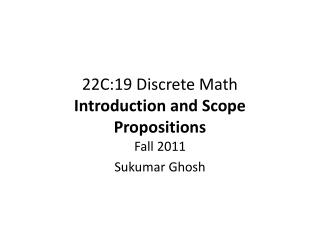DownloadDownload Presentation22C:19 Discrete Math Introduction and Scope Propositions

# 22C:19 Discrete Math Introduction and Scope Propositions

Download Presentation## 22C:19 Discrete Math Introduction and Scope Propositions

- - - - - - - - - - - - - - - - - - - - - - - - - - - E N D - - - - - - - - - - - - - - - - - - - - - - - - - - -
##### Presentation Transcript

1. 22C:19 Discrete MathIntroduction and ScopePropositions Fall 2011 Sukumar Ghosh

2. The Scope Discrete mathematicsstudies mathematical structures that are fundamentally discrete,not supporting or requiring the notion of continuity(Wikipedia). Deals with countable things.

3. Why Discrete Math? Discrete math forms the basis for computer science: • Sequences • Digital logic (how computers compute) • Algorithms • Program correctness • Probability and gambling (or taking risks) “Continuous” math forms the basis for most physical and biological sciences

4. Propositions A proposition is a statement that is either true or false “The sky is blue” “Today the temperature is below freezing” “9 + 3 = 12” Not propositions: “Who is Bob?” “How many persons are there in this group?” “X + 1 = 7.”

5. Propositional (or Boolean) variables These are variables that refer to propositions. • Usually denoted by lower case letters p, q, r, s, etc. • Each can have one of two values true (T) or false (F) A proposition can be: • A single variable p • A formula of multiple variables like p∧ q, s∨¬r)

6. Propositional (or Boolean) operators

7. Logical operator: NOT

8. Logical operator: AND

9. Logical operator: OR

10. Logical operator: EXCLUSIVE OR Note. p ⊕ q is false if both p, q are true or both are false

11. (Inclusive) OR or EXCLUSIVE OR?

12. Logical Operator NAND and NOR

13. Conditional Operator

14. Conditional operators

15. Conditional operators

16. Set representations A proposition p can also be represented by a set (a collection of elements) for which the proposition is true. Universe p p p p ∧ q p q q p ∨ q ¬p Venn diagram

17. Bi-conditional Statements

18. Translating into English

19. Translating into English Great for developing intuition about propositional operators. You can access the Internet from campus (p) only if you are a CS major (cs), or you are not a freshman (f) p ⟶ (cs∨¬f)

20. Precedence of Operators

21. Boolean operators in search

22. Tautology and Contradiction

23. Equivalence

24. Examples of Equivalence

25. Examples of Equivalence

26. More Equivalences Associative Laws DistributiveLaw Law of absorption

27. De Morgan’s Law You can take 22C:21 if you take 22C:16 and 22M:26 You cannot take 22C:21 if you have not taken 22C:16 or 22M:26

28. How to prove Equivalences Examples? Follow class lectures.

29. Muddy Children Puzzle A father tells his two children, a boy and a girl, to play in the backyard without getting dirty. While playing, both children get mud on their foreheads. After they returned home, the father said: “at least one of you has a muddy forehead,” and then asked the children to answer YES or NO to the question: “Do you know if you have a muddy forehead?” the father asked the question twice. How will the children answer each time?

30. Wrap up Understand propositions, logical operators and their usage. Understand equivalence, tautology, and contradictions. Practice proving equivalences, tautology, and contradictions. Study the Muddy Children Puzzle from the book.